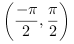Scilab Home page | Wiki | Bug tracker | Forge | Mailing list archives | ATOMS | File exchange
Change language to: English - Português - 日本語 - Русский

See the recommended documentation of this function

# atan

### Calling Sequence

```phi = atan(x)
phi = atan(y, x)```

### Arguments

x

a real or complex scalar, vector or matrix.

phi

a real or complex scalar, vector or matrix.

x, y

a real scalars, vectors or matrices of the same size.

phi

a real scalar, vector or matrix.

### Description

The first form computes the 2-quadrant inverse tangent, which is the inverse of `tan(phi)`. For real `x`, `phi` is in the interval. For complex `x`, `atan` has two singular, branching points `+%i`, `-%i` and the chosen branch cuts are the two imaginary half-straight linesand.

The second form computes the 4-quadrant arctangent (`atan2` in Fortran), this is, it returns the argument (angle) of the complex number `x+i*y`. The range of `atan(y, x)` is.

For real arguments, both forms yield identical values if `x>0`.

In case of vector or matrix arguments, the evaluation is done element-wise, so that `phi` is a vector or matrix of the same size with `phi(i, j) = atan(x(i, j))` or `phi(i,j) = atan(y(i, j), x(i, j))`.

### Examples

```// examples with the second form
x=[1,%i,-1,%i]
phase_x=atan(imag(x),real(x))
atan(0,-1)
atan(-%eps,-1)

// branch cuts
atan(-%eps + 2*%i)
atan(+%eps + 2*%i)
atan(-%eps - 2*%i)
atan(+%eps - 2*%i)

// values at the branching points
ieee(2)
atan(%i)
atan(-%i)```

• tan — tangente
• unwrap — unwrap a Y(x) profile or a Z(x,y) surface. Unfold a Y(x) profile
• ieee — détermine le mode d'exception IEEE pour les calculs